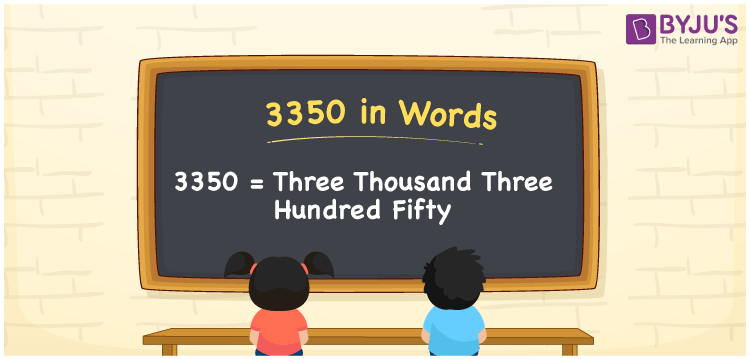# 3350 in Words

3350 in words is written as “Three Thousand Three Hundred Fifty”. In Maths, 3350 is a cardinal number that expresses a quantity or a value. 3350 can also be expressed as 3000 + 350. To write the number 3350 in words, we have used here place value chart. An example is “If the price of a shoe rack is Rs.3350, then we can write the amount as Rupees Three Thousand Three Hundred Fifty, in English. Learn more about Numbers In Words at BYJU’S.

 3350 in Words Three Thousand Three Hundred Fifty Three Thousand Three Hundred Fifty in Numbers 3350

## 3350 in English Words## How to Write 3350 in Words?

The number 3350 in words can be written using a place value system, where we can identify the position of each digit. Since 3350 is a four-digit number, thus the place value of the digits is given by:

 Thousands Hundreds Tens Ones 3 3 5 0

From the above table,

3 → Thousands place

3 → Hundreds place

5 → Tens

0 → Ones place

Hence, when we read the number from right to left, it is Three Thousand Three Hundred Fifty.

### Expanded Form of 3350

We can write the expanded form as:

3 x Thousand + 3 × Hundred + 5 × Ten + 0 × One

= 3 x 1000 + 3 × 100 + 5 × 10 + 0 × 1

= 3000 + 300 + 50 + 0

= Three Thousand Three Hundred Fifty

3350 is a whole number that is succeeded by 3349 and preceded by 3351. Learn more about the number 3350 below:

• 3350 in Words – Three Thousand Three Hundred Fifty
• Is 3350 an odd number? – No
• Is 3350 an even number? – Yes
• Is 3350 a perfect square number? – No
• Is 3350 a perfect cube number? – No
• Is 3350 a prime number? – No
• Is 3350 a composite number? – Yes

## Frequently Asked Questions on 3350 in words

Q1

### What is 3350 in words?

3350 in words is given by Three Thousand Three Hundred Fifty.
Q2

### What is the rule to write 3350 in words?

3350 in words is written based on the place values of each digit. 3 is at thousands,3  at hundreds, 5 at tens and 0 at ones. Thus, reading from right to left, it is Three Thousand Three Hundred Fifty.
Q3

### What is the value of 3350 plus 200 in words?

3350 + 200 = 3550, i.e., Three Thousand Five Hundred Fifty in words